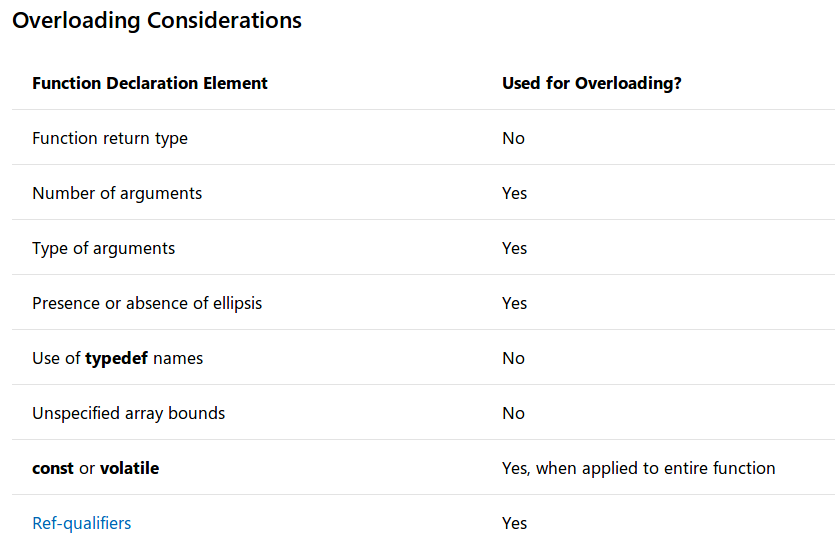# [c++11]函数¶

## 函数类别¶

• 成员函数（member function）：定义在类作用域内
• 自由函数（free function）：定义在命名空间作用域或者全局作用域内，也称为非成员函数（non-member function

## 函数声明¶

return_type function_name(parameter list);

• 返回类型指定了函数返回值的类型，设为void表示无返回值。从c++11开始，可以使用auto作为返回类型，编译器会自动推导返回值的类型
• 函数名必须以字母或下划线开头，不能包含空格。通常标准库函数名中的前缀下划线表示私有成员函数，或者表示不希望用户使用的非成员函数
• 参数列表是由零个或多个参数组成，用逗号分隔的集合。用于指定类型和可选的本地名称，通过该名称可以在函数体内部访问

### 可选说明符¶

1. constexpr：表明函数的返回值是一个常量，可以在编译时计算

constexpr float exp(float x, int n);

2. externstatic：影响其链接规范。声明为extern表明其拥有外部链接；声明为static表明其拥有内部链接。参考链接

3. inline：设置为内联函数。它指示编译器用函数代码本身替换对函数的每个调用。在函数执行速度快并且在性能关键的代码部分反复调用的情况下，内联可以帮助提高性能

inline double Account::GetBalance()
{
return balance;
}

4. noexcept：是否抛出异常，指定函数是否可以引发异常

5. cv限定符：仅适用于成员函数，指定函数是否是const或者volatile
6. virtual，override和final：仅适用于成员函数。virtual指定函数可在派生类中重写；override指定函数是重写父类的virtual函数；final指定函数不能被后续的派生类重写
7. static：仅适用于成员函数，表明该函数不与任一个对象相关联
8. 引用限定符：仅适用于非成员函数，它向编译器指定当隐式对象参数（*this）是右值引用而不是左值引用时要选择的函数重载

## 函数定义¶

return_type function_name(parameter list) {
。。。
。。。
}


## const和constexpr¶

class CTest {

public:
int f(int a) const;

private:
int b = 4;
};

int CTest::f(int a) const {
b = a;

return a + b;
}

int main() {
CTest c;
cout << c.f(3) << endl;
}


/home/zj/CLionProjects/first/main.cpp: In member function ‘int CTest::f(int) const’:
/home/zj/CLionProjects/first/main.cpp:18:7: error: assignment of member ‘CTest::b’ in read-only object
b = a;
^
CMakeFiles/first.dir/build.make:62: recipe for target 'CMakeFiles/first.dir/main.cpp.o' failed


constexpr auto int f(int a) {
return a + 3;
}


## 函数重载¶### 参数匹配¶

• 找到完全匹配的参数列表
• 执行了一个简单的转换
• 实现了整数提升
• 存在到所需参数类型的标准转换
• 存在到所需参数类型的用户定义转换
• 找到省略号表示的参数

### 参数类型差异¶

• 给定类型的参数和对该类型的引用被认为是相同的
• constvolatile声明的参数类型和原始参数类型被认为相同的

### 函数重载限制¶

1. 重载函数集中任意两个函数都拥有不同的参数列表
2. 重载具有相同类型参数列表的函数（仅基于返回类型）是错误的
3. 成员函数不能仅基于一个静态函数和另一个非静态函数而重载
4. typedef声明不定义新类型；它们引入了现有类型的同义词。它们不影响重载机制
5. 枚举类型是不同的类型，可用于区分重载函数
6. 为区分重载函数，类型array ofpointer to被认为是相同的，但仅适用于单维数组 void Print(char *szToPrint) { cout << szToPrint << endl; } // error, redefinition void Print(char szToPrint[]) { cout << szToPrint << endl; } 对于多维数组，从第二维开始的后续维度被认为是类型的一部分，所以可用于区分重载函数  void Print(char szToPrint[]) { cout << szToPrint << endl; }

void Print(char szToPrint[]) { cout << szToPrint << endl; }

void Print(char szToPrint[]) { cout << szToPrint << endl; } 

## 函数模板¶

template<typename Lhs, typename Rhs>
auto Add2(const Lhs& lhs, const Rhs& rhs)
{
return lhs + rhs;
}


## 函数参数¶

### 可变参数¶

C++支持函数可变参数，类似C语言中的printf函数

C stdarg.h的使用

Functions with Variable Argument Lists (C++)

### 引用参数¶

void DoSomething(std::string& input){...}


void DoSomething(const std::string& input){...}


c++11规定：要显式处理由右值引用（rvalue-reference）或左值引用（lvalue-reference）传递的参数，请在参数上使用双和号（double-ampersand）来表示通用引用：

void DoSomething(const std::string&& input){...}


### void¶

void f()           # 正确
void f(void)       # 正确
void f(void, void) # error


void类型派生的类型（例如指向void的指针和void数组）可以出现在参数声明列表的任何位置

int dd(void *a) {
return *static_cast<int *>(a);
}

int main() {
int a = 3;
cout << dd(&a) << endl;
}


### 默认参数¶

void def(int a, float b = 3.21, char c = '2') {
cout << a << " ";
cout << b << " ";
cout << c << endl;
}

int main() {
def(1);
def(1, 32.11);
def(1, 3.33, 'a');
}


1 3.21 2
1 32.11 2
1 3.33 a


## 返回类型¶

auto def(int a, float b = 3.21, char c = '2') -> int {
return b;
}

int main() {
cout << def(1) << endl;
cout << def(1, 32.11) << endl;
cout << def(1, 3.33, 'a') << endl;
}


3
32
3


auto def(int a, float b = 3.21, char c = '2') -> decltype(a) {


## 函数局部变量¶

auto def(float b) {
static float ff;
cout << ff << endl;
ff = b;
}

int main() {
def(3);
def(3.32);
def(3.2221);
}


0
3
3.32


## Overloading，overriding和hiding¶

• 如果派生类函数声明了基类virtual函数，则进行了重写（override）操作
• 如果基类函数没有声明为virtual，那么派生类函数隐藏（hide）了它。重写（overridding）和隐藏（hiding）操作不同于重载（overloaded

### 局部函数¶

void func(int i) {
cout << "Called file-scoped func : " << i << endl;
}

void func(char *sz) {
cout << "Called locally declared func : " << sz << endl;
}

int main() {
// Declare func local to main.
extern void func(char *sz);

//    func(3);   // C2664 Error. func( int ) is hidden.
func("s");
}


main函数内部的func函数声明隐藏了外部func函数重载，所以只能支持输入字符数组的参数

// declaration_matching2.cpp
class Account
{
public:
Account()
{
}
double Deposit( double dAmount, char *szPassword );

private:
double Deposit( double dAmount )
{
return 0.0;
}
int Validate( char *szPassword )
{
return 0;
}

};

int main()
{
// Allocate a new object of type Account.
Account *pAcct = new Account;

// Deposit $57.22. Error: calls a private function. // pAcct->Deposit( 57.22 ); // Deposit$57.22 and supply a password. OK: calls a
//  public function.
pAcct->Deposit( 52.77, "pswd" );
}

double Account::Deposit( double dAmount, char *szPassword )
{
if ( Validate( szPassword ) )
return Deposit( dAmount );
else
return 0.0;
}


## 内联函数¶

class Account
{
public:
Account(double initial_balance) { balance = initial_balance; }
double GetBalance();
private:
double balance;
};

inline double Account::GetBalance()
{
return balance;
}